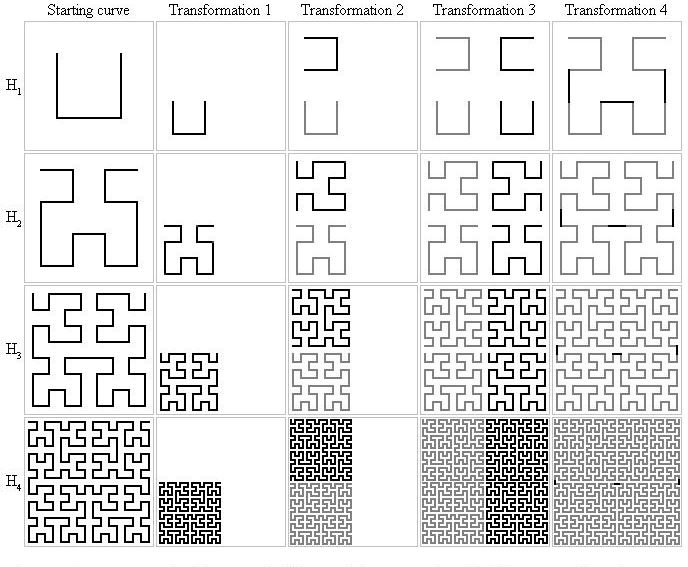Online JudgeProblem SetAuthorsOnline ContestsUser
Web Board
F.A.Qs
Statistical Charts
Problems
Submit Problem
Online Status
Prob.ID:
Register
Authors ranklist
Current Contest
Past Contests
Scheduled Contests
Award Contest
Register
Language:
Hilbert Curve Intersections
 Time Limit: 1000MS Memory Limit: 10000K Total Submissions: 371 Accepted: 96

DescriptionDavid Hilbert proved the existence of a very counter-intuitive curve that fills space. The construction of the Hilbert curve is based on a sequence of curves, H1, H2, H3, H4, ... composed of horizontal and vertical segments. Each curve lies in the unit square [0, 1] * [0, 1]. H1 contains just three segments, connecting the points (1/4, 3/4) to (1/4, 1/4) to (3/4, 1/4) to (3/4, 3/4). Hn is defined recursively in terms of Hn-1, for n = 2, 3, ... by four transformations:

1.Halve all coordinates in Hn-1.
2.Add a copy rotated 90 degrees counterclockwise about the point (0, 1/2).
3.Add the reflection across the line x = 1/2.
4.Let m = 1/2n+1. Add segments connecting endpoints (1/2 - m, 1/2 - m) to (1/2 + m, 1/2 - m), (m, 1/2 - m) to (m, 1/2 + m), and (1 - m, 1/2 - m) to (1 - m, 1/2 + m).
Your job is to count the number of intersections of horizontal line segments with these curves. For example, consider Figures 1 and 2, which illustrate the first two example input data sets below.The coordinates of vertices of Hn are odd multiples of 1/2n+1. The coordinates of horizontal segment endpoints will always be multiples of 1/2n. Hence the specified horizontal segment can only cross vertical segments in Hn.

Input

Input consists of one to 100 data sets, one per line, followed by a final line containing only 0. Each data set consists of four integers separated by blanks in the form
n x1 x2 y
which represents Hn and the segment from (x1/2n, y/2n) to (x2/2n, y/2n), where 0 < n < 31, x1 < x2, and each of x1, x2, and y lie in the range 0 to 2n, inclusive.

Output

The output is one integer per line for each data set: the number of intersections of Hn with the segment. Caution: A brute force solution that computes each intersection individually will not finish within the one minute time limit. As you can see below, there may be more than one billion intersections for any data set.

Sample Input

```3 2 7 7
4 0 16 1
30 1 1073741823 1
0```

Sample Output

```3
16
1073741822```

Source

[Submit]   [Go Back]   [Status]   [Discuss]Home PageGo BackTo top